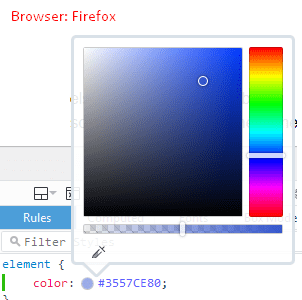# What is the HEX code for Transparent color?

There is not a Transparent color code, but there is an Opacity styling. Opacity is the degree to which content behind an element is hidden, and is the opposite of transparency.

## Alpha Component

Transparency is a property outside the color itself, and it's also known as alpha component, You can't code transparency as pure RGB (Red-Green-Blue), but you can use the RGBA notation, in which you define the color + alpha channel together:

rgba(red, green, blue, alpha)

Where alpha defines the opacity as a number between 0.0 (fully transparent) and 1.0 (fully opaque)

Example:
color: rgba(255, 0, 0, 0.5); /* 0.5 is the transparency value */The transparency value 0.5 is more like semi-transparent, changing the value from 0.5 to 0 gave you true transparency. Also, note that using RGBA over opacity means that your child elements are not transparent.

If you want "transparent", just set the last number to 0, regardless of the color. All of the following result in "transparent" even though the color part is set to 100% red, green and blue respectively:

color: rgba(255, 0, 0, 0); /* red, transparent */ color: rgba(0, 255, 0, 0); /* blue, transparent */ color: rgba(0, 0, 255, 0); /* green, transparent */

## HEX code for Transparent color

There's also the HEXA notation ( #RRGGBBAA ) now supported on all major browsers, which is pretty much the same as RGBA but using hexadecimal notation instead of decimal. It's called HEXA ( HEX + Alpha ). It takes in 8 digits instead of 6. The last pair is Alpha. So the pattern of pairs is #RRGGBBAA. Having 4 digits also works: #RGBA.

color: #FF000080; /* red at 50% opacity */

Here the FF0000 is the color and 80 is the transparency. Where 80 is the hexadecimal equivalent of 50%.

For the most part, Chrome and Firefox have started supporting this:## HEX values for opacity

Following is a correct table of percentages to hex values for opacity. To think in terms of transparency instead, flip the order of the percentages (more opaque = less transparent).

% Hex
100% FF
95% F2
90% E6
85% D9
80% CC
75% BF
70% B3
65% A6
60% 99
55% 8C
50% 80
45% 73
40% 66
35% 59
30% 4D
25% 40
20% 33
15% 26
10% 1A
5% 0D
0% 00

## How is it calculated?

Percentage to hex values
1. decimal = percentage * 255 / 100 . ex : decimal = 50*255/100 = 127.5
2. convert decimal to hexadecimal value . ex: 127.5 in decimal = 7*16ˆ1 + 15 = 7F in hexadecimal
Hex values to percentage
1. convert the hexadecimal value to decimal. ex: D6 = 13*16ˆ1 + 6 = 214
2. percentage = (value in decimal ) * 100 / 255. ex : 214 *100/255 = 84%